# 小白学 Python 数据分析（17）：Matplotlib（二）基础操作

2019/04/10 10:10## 坐标轴标题设置import matplotlib.pyplot as plt

x_data = ['2011','2012','2013','2014','2015','2016','2017']
y_data = [58000,60200,63000,71000,84000,90500,107000]

plt.xlabel('年份')
plt.ylabel('销量')

plt.plot(x_data, y_data)

plt.show()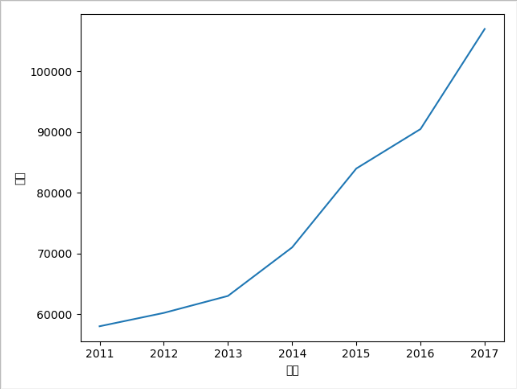RuntimeWarning: Glyph 24180 missing from current font.


plt.rcParams['font.sans-serif']=['SimHei']


import matplotlib.pyplot as plt

plt.rcParams['font.sans-serif']=['SimHei']

x_data = ['2011','2012','2013','2014','2015','2016','2017']
y_data = [58000,60200,63000,71000,84000,90500,107000]

plt.xlabel('年份')
plt.ylabel('销量')

plt.plot(x_data, y_data)

plt.show()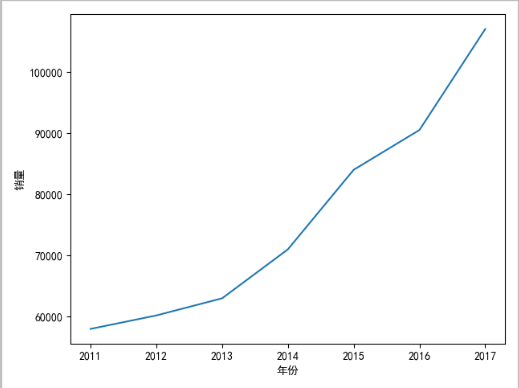plt.xlabel('年份', labelpad=50)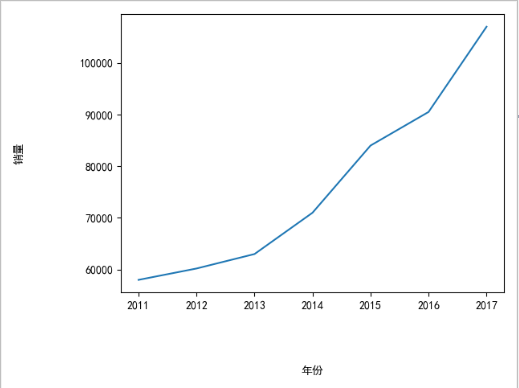plt.xlabel('年份', labelpad=50, fontsize='xx-large', fontweight='bold', rotation='vertical', backgroundcolor='red')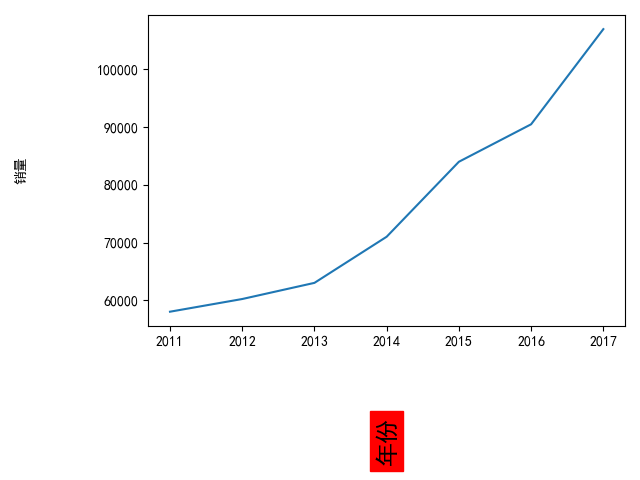xlabel 中常用的一些参数：

• fontsize : 设置字体大小，默认12，可选参数 ['xx-small', 'x-small', 'small', 'medium', 'large','x-large', 'xx-large']
• fontweight : 设置字体粗细，可选参数 ['light', 'normal', 'medium', 'semibold', 'bold', 'heavy', 'black']
• fontstyle : 设置字体类型，可选参数[ 'normal' | 'italic' | 'oblique' ]，italic斜体，oblique倾斜
• verticalalignment : 设置水平对齐方式 ，可选参数 ： 'center' , 'top' , 'bottom' ,'baseline'
• horizontalalignment : 设置垂直对齐方式，可选参数：left,right,center
• rotation : (旋转角度)可选参数为:vertical,horizontal 也可以为数字
• alpha : 透明度，参数值0至1之间
• backgroundcolor : 标题背景颜色
• bbox : 给标题增加外框 ，常用参数如下：
• boxstyle 方框外形
• facecolor (简写fc)背景颜色
• edgecolor (简写ec)边框线条颜色
• edgewidth 边框线条大小

## 刻度设置

import matplotlib.pyplot as plt

plt.rcParams['font.sans-serif']=['SimHei']

x_data = [2011,2012,2013,2014,2015,2016,2017]
y_data = [58000,60200,63000,71000,84000,90500,107000]

plt.xticks(x_data, ['2011年','2012年','2013年','2014年','2015年','2016年','2017年'])
plt.yticks(y_data)

plt.plot(x_data, y_data)

plt.show()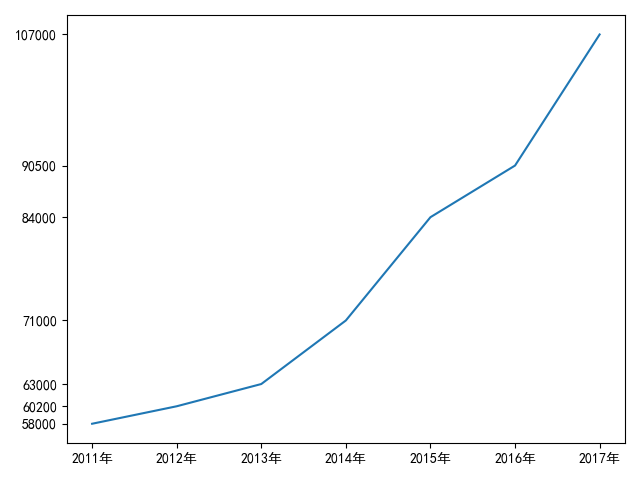plt.xticks(x_data, [])
plt.yticks(y_data, [])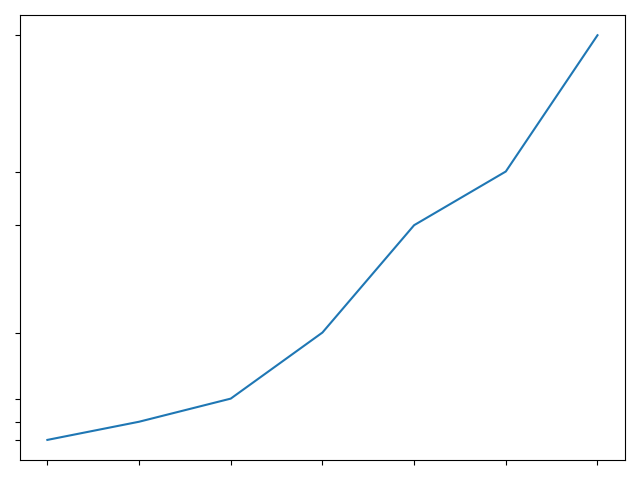plt.axis("off")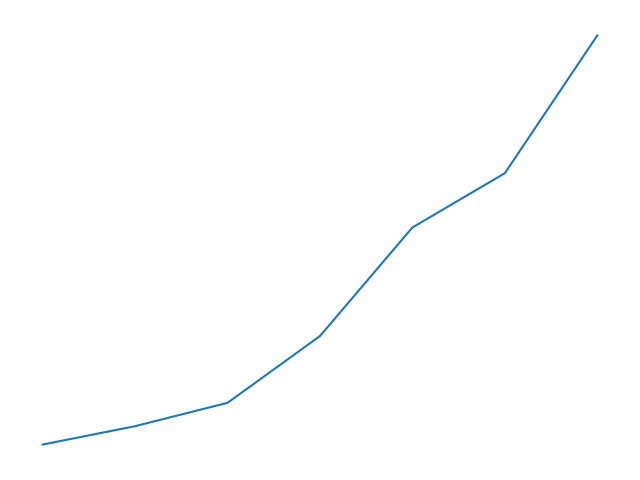## 范围设置

import matplotlib.pyplot as plt

plt.rcParams['font.sans-serif']=['SimHei']

x_data = [2011,2012,2013,2014,2015,2016,2017]
y_data = [58000,60200,63000,71000,84000,90500,107000]

plt.xlim(2011, 2020)
plt.ylim(50000, 90000)

plt.plot(x_data, y_data)

plt.show()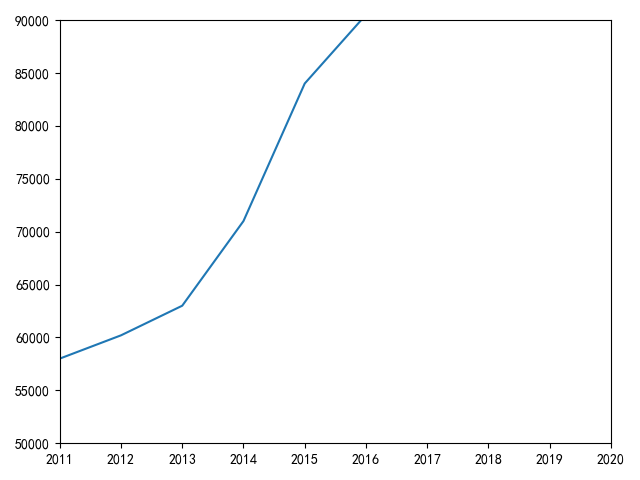## 网格线设置

import matplotlib.pyplot as plt

plt.rcParams['font.sans-serif']=['SimHei']

x_data = [2011,2012,2013,2014,2015,2016,2017]
y_data = [58000,60200,63000,71000,84000,90500,107000]

plt.plot(x_data, y_data)

plt.grid(b=True)

plt.show()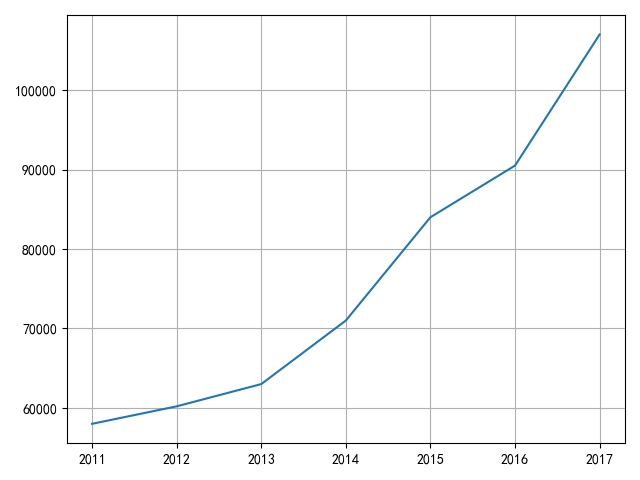# 开启 x 轴网格线
plt.grid(b=True, axis='x')
# 开启 y 轴网格线
plt.grid(b=True, axis='y')


## 图例设置

import matplotlib.pyplot as plt

plt.rcParams['font.sans-serif']=['SimHei']

x_data = [2011,2012,2013,2014,2015,2016,2017]
y_data = [58000,60200,63000,71000,84000,90500,107000]

plt.plot(x_data, y_data, label = '折线图')
plt.bar(x_data, y_data, label = '柱状图')

plt.legend()

plt.show()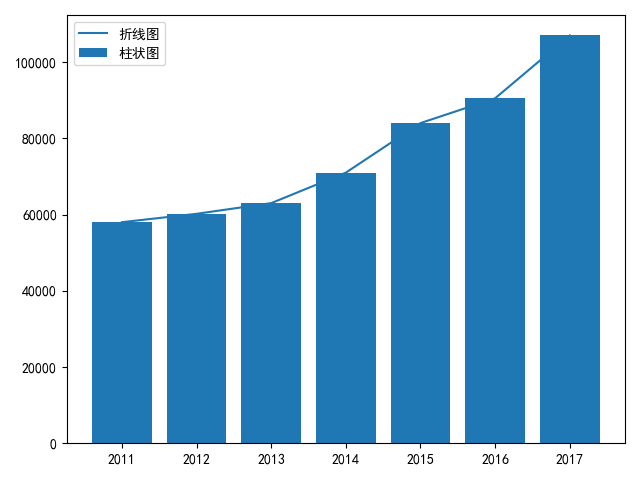## 图表标题设置

plt.title(label='xxx 公司 xxx 产品销量')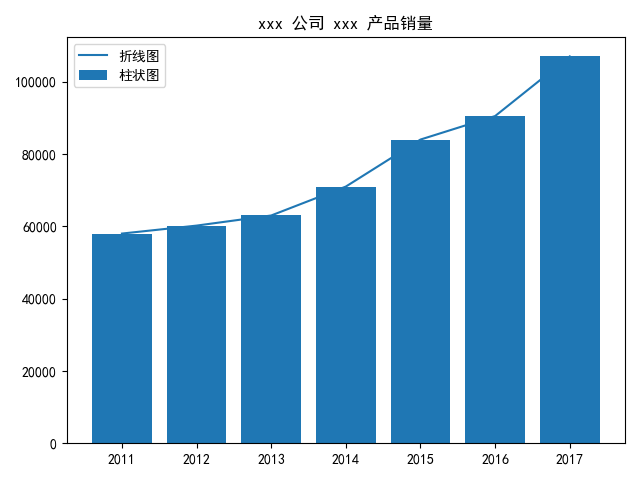## 参考

https://blog.csdn.net/The_Time_Runner/article/details/89927708

0
0 收藏

### 作者的其它热门文章0 评论
0 收藏
0